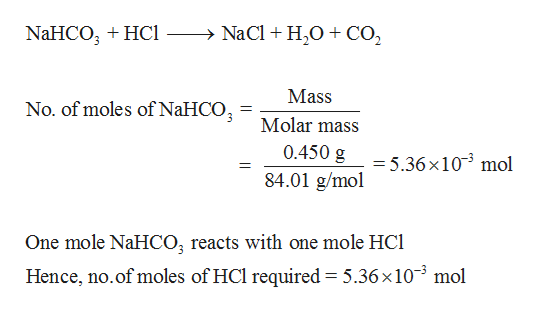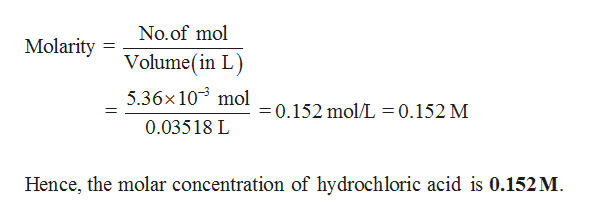# How do I calculate the molar concentration of hydrochloric acid if 35.18 of hydrochloric acid was required to neutralize .450g of sodium bicarbonate to a phenolphathalein end point Then calculate mass percent concentration of hydrochloric acid using the molar concentration calculated in previous question and assuming density is 1.01 g

Question
55 views

How do I calculate the molar concentration of hydrochloric acid if 35.18 of hydrochloric acid was required to neutralize .450g of sodium bicarbonate to a phenolphathalein end point

Then calculate mass percent concentration of hydrochloric acid using the molar concentration calculated in previous question and assuming density is 1.01 g

check_circle

Step 1

Number of moles of HCl required to neutralize 0.450g of sodium bicarbonate is calculated,help_outlineImage TranscriptioncloseNaCl H20CO2 NaHCO HC Mass No. of moles of NaHCO Molar mass 0.450 g = 5.36x103 mol 84.01 g/mol One mole NaHCO; reacts with one mole HC Hence, no.of moles of HCl required = 5.36 x 103 mol fullscreen
Step 2

Volume of HCl is 35.18 mL = 0.03518 L (1 mL = 0.001L).

Molar conce...help_outlineImage TranscriptioncloseNo.of mol Molarity Volume(in L 5.36x 103 mol 0.152 mol/L=0.152 M 0.03518 L Hence, the molar concentration of hydrochloric acid is 0.152M. fullscreen

### Want to see the full answer?

See Solution

#### Want to see this answer and more?

Solutions are written by subject experts who are available 24/7. Questions are typically answered within 1 hour.*

See Solution
*Response times may vary by subject and question.
Tagged in

### Quantitative analysis